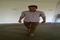Join the social network of Tech Nerds, increase skill rank, get work, manage projects...

• # SQL Database@mukesh.tomar• 0
• 1
• 0
• 0
• 0
• 0
• 0
• 0
• 108
Comment on it

SQL follow the unique set of rules that is called syntax ,follow the rules given below
SQL database is not a case sensitive . Generally in SQL database keywords are written in uppercase SQL statements are dependent on text lines .
You can do task in a database with SQL statements .
SQL depends on relational algebra .
What is SQL statement ?
SQL statements is started with any of the SQL commands/keywords like select , insert , update , alter etc and that statement should be end with semicolon .
Example of SQL statement :

```select **column-name** from **table-name**
```

In this semicolon indicate the end of statement .
SQL database support various data types , list is given below
integer -> For integer number .
smallint -> For small integer number .
Numeric -> Numeric ( p , s ) where p is precision and s is scale value .
real -> single precision floating point number .
decimal -> Decimal ( p , s ) where p is precision and s is scale value .
double precision -> Double precision floating point number .
float -> * Float (P) where p is precision .
*
character ->
char ( x ) where x is the character number to store .
character varying -> varchar2(x) where x is the character number to store .
Bit -> Bit ( x ) where x is the number of bits to store .
Date -> It is used to store year, month and days values .
Time -> It is used to store hour , month , day , hour , minute and second values . etc

SQL Arithmetic operator -> + operator -> It is used to add both the operands .
Example ->

```If a=10 and b=5 then a + b = 15 ;
```

- opertor -> It is used to subtract both the operands .
Example ->

```If a=10 and b=5 then a - b = 5 ;
```

* opertor -> It is used to multiply both the operands .
Example ->

```If a=10 and b=5 then a * b =50 ;
```

/ opertor -> It is used to divide both the operands .
Example ->

```If a=10 and b=5 then a / b = 2 ;
```

% opertor -> It divide left hand operand by right hand operand and return the remainder .
Example ->

```If a=10 and b=5 then a % b = 0 ;
```

## 0 Comment(s)

OR
OR
Register

OR
Fill out the form below and instructions to reset your password will be emailed to you:

• Hire
• Post Projects

### Post Projects

• All at 0 Cost ....
• Post Tech Job
• Select Best Bidder
• Track the Project
• Approve Work and Pay safely
• Browse Nerds
• Work
• Find Projects Find Projects
• UI Design and UX
• Software Engineering
View more...
View less...
• Marketing
• General
View more...
View less...
• Manage
• Company Company

### Manage Company

• All at 0 Cost ....
• Manage Company and Employee Profiles
• Company wide Employee Productivity Reports
• Knowledge Sharing and Collaboration Tools
• Get Sales Lead and Bid for Tech Projects
• Send Invoices and Receive Payment Safely
• Learn
• Nerd Digest Nerd Digest
• UI Design and UX
• Software Engineering
View more...
View less...
• Marketing
• General
View more...
View less...
• Tech Q & A Tech Q & A
• UI Design and UX
• Software Engineering
View more...
View less...
• Marketing
• General
View more...
View less...